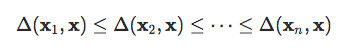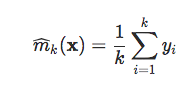{{announcement.body}}
{{announcement.title}}

# Foundations of Machine Learning: Part 1

DZone 's Guide to

# Foundations of Machine Learning: Part 1

### In this post, we take a look at the basics of what exactly it takes to make a machine 'learn,' and the history of the concept.

· AI Zone ·
Free Resource

Comment (0)

Save
{{ articles.views | formatCount}} ViewsThis post is the fifth one of our series on the history and foundations of econometric and machine learning models. The first four were on econometrics techniques. Part 4 is online here.

In parallel with these tools developed by and for economists, a whole literature has been developed on similar issues, centered on the problems of prediction and forecasting. For Breiman (2001a), the first difference comes from the fact that statistics has developed around the principle of inference (or to explain the relationship linking y to variables x) while another culture is primarily interested in prediction. In a discussion that follows the article, David Cox states very clearly that in statistics (and econometrics) "predictive success... is not the primary basis for model choice ". We will get back here on the roots of automatic learning techniques. The important point, as we will see, is that the main concern of machine learning is related to the generalization properties of a model, i.e. its performance - according to a criterion chosen a priori - on new data, and therefore on non-sample tests.

## A Learning Machine

Today, we speak of "machine learning" to describe a whole set of techniques, often computational, as alternatives to the classical econometric approach. Before characterizing them as much as possible, it should be noted that, historically, other names have been given. For example, Friedman (1997) proposes to make the link between statistics (which closely resemble econometric techniques — hypothesis testing, ANOVA, linear regression, logistics, GLM, etc.) and what was then called "data mining" (which then included decision trees, methods from the closest neighbours, neural networks, etc.). The bridge between those two cultures corresponds to "statistical learning" techniques described in Hastie et al (2009). But one should keep in mind that machine learning is a very large field of research.

The so-called "natural" learning (as opposed to machine learning) is that of children, who learn to speak, read and play. Learning to speak means segmenting and categorizing sounds, and associating them with meanings. A child also learns simultaneously the structure of his or her mother tongue and acquires a set of words describing the world around him or her. Several techniques are possible, ranging from rote learning, generalization, discovery, more or less supervised or autonomous learning, etc. The idea in artificial intelligence is to take inspiration from the functioning of the brain to learn, to allow "artificial" or "automatic" learning, by a machine. The first application was to teach a machine to play a game (tic-tac-toe, chess, Go, etc.). An essential step is to explain the objective it must achieve to win. One historical approach has been to teach the machine the rules of the game. If it allows you to play, it will not help the machine to play well. Assuming that the machine knows the rules of the game, and that it has a choice between several dozen possible moves, which one should it choose? The classical approach in artificial intelligence uses the so-called min-max algorithm using an evaluation function: in this algorithm, the machine searches forward in the possible moves tree, as far as the calculation resources allow (about ten moves in chess, for example). Then, it calculates different criteria (which have been previously indicated) for all positions (number of pieces taken, or lost, occupancy of the center, etc. in our example of the chess game), and finally, the machine plays the move that allows it to maximize its gain. Another example may be the classification and recognition of images or shapes. For example, the machine must identify a handwritten number (checks, ZIP codes on envelopes, etc). It is a question of predicting the value of a variable, y, knowing that a priori ∈{0,1,2,⋯,8,9}. A classical strategy is to provide the machine with learning bases, in other words here millions of labelled (identified) images of handwritten numbers. A simple (and natural) strategy is to use a decision criterion based on the closest neighbors whose labels are known (using a predefined metric).

The method of the closest neighbors ("k-nearest neighbors") can be described as follows: we consider (as in the previous part) a set of n observations, i. e. pairs (yixi) with x∈ Rp. Let us consider a distance Δ on Rp
(the Euclidean distance or the Mahalanobis distance, for example). Given a new observation ∈ Rp, let us assume the ordered observations as a function of the distance between the xand x, in the sense thatthen we can consider as prediction for y the average of the nearest k neighbours,Learning here works by induction, based on a sample (called the learning - or training - sample).

Automatic learning includes those algorithms that give computers the ability to learn without being explicitly programmed (as Arthur Samuel defined it in 1959). The machine will then explore the data with a specific objective (such as searching for the nearest neighbours in the example just described). Tom Mitchell proposed a more precise definition in 1998: a computer program is said to learn from experience E in relation to a task T and a performance measure P, if its performance on T, measured by P, improves with experience E. Task T can be a defect score for example, and performance P can be the percentage of errors made. The system learns if the percentage of predicted defects increases with experience.

As we can see, machine learning is basically a problem of optimizing a criterion based on data (from now on called learning). Many textbooks on machine learning techniques propose algorithms, without ever mentioning any probabilistic model. In Watt et al (2016) for example, the word "probability" is mentioned only once, with this footnote that will surprise and make any econometrician smile: "the logistic regression can also be interpreted from a probabilistic perspective" (page 86). But many recent books offer a review of machine learning approaches using probabilistic theories, following the work of Vaillant and Vapnik. By proposing the paradigm of "probably almost correct" learning (PAC), a probabilistic flavor has been added to the previously very computational approach, by quantifying the error of the learning algorithm (usually in a classification problem).

Topics:
machine learning ,foundations of ml ,ai ,artifical intelligence

Comment (0)

Save
{{ articles.views | formatCount}} Views

Published at DZone with permission of

Opinions expressed by DZone contributors are their own.